# ML Aggarwal Class 10 Solutions for ICSE Maths Chapter 17 Mensuration Ex 17.2

## ML Aggarwal Class 10 Solutions for ICSE Maths Chapter 17 Mensuration Ex 17.2

ML Aggarwal Class 10 Solutions for ICSE Maths Chapter 17 Mensuration Ex 17.2

Take π = $$\\ \frac { 22 }{ 7 }$$ unless stated otherwise.

Question 1.
Write whether the following statements are true or false. Justify your answer.
(i) If the radius of a right circular cone is halved and its height is doubled, the volume will remain unchanged.
(ii) A cylinder and a right circular cone are having the same base radius and same height. The volume of the cylinder is three times the volume of the cone.
(iii) In a right circular cone, height, radius and slant height are always the sides of a right triangle.
Solution:LearnCram.com also covered previous Year argumentative essay topics asked in ICSE board exams.

Question 2.
Find the curved surface area of a right circular cone whose slant height is 10 cm and base radius is 7 cm.
Solution: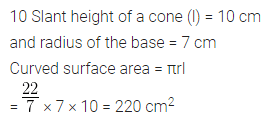Question 3.
Diameter of the base of a cone is 10.5 cm and slant height is 10 cm. Find its curved surface area.
Solution: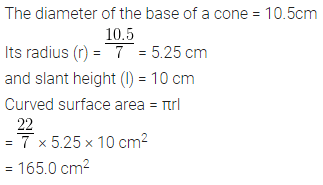Question 4.
Curved surface area of a cone is 308 cm2 and its slant height is 14 cm. Find
(ii) total surface area of the cone.
Solution:Question 5.
Find the volume of the right circular cone with
(i) radius 6 cm and height 7 cm
(ii) radius 3.5 cm and height 12 cm.
Solution: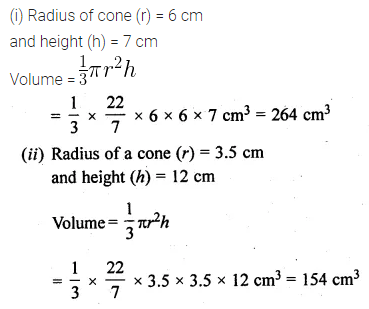Question 6.
Find the capacity in litres of a conical vessel with
(i) radius 7 cm, slant height 25 cm
(ii) height 12 cm, slant height 13 cm
Solution: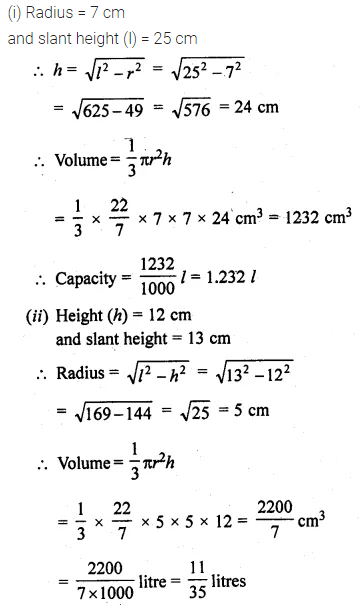Question 7.
A conical pit of top diameter 3.5 m is 12 m deep. What is its capacity in kiloliters?
Solution:Question 8.
If the volume of a right circular cone of height 9 cm is 48π cm3, find the diameter of its base.
Solution:Question 9.
The height of a cone is 15 cm. If its volume is 1570 cm3, find the radius of the base. (Use π = 3.14)
Solution:Question 10.
The slant height and a base diameter of a conical tomb are 25 m and 14 m respectively. Find the cost of whitewashing its curved surface area at the rate of Rs 210 per 100 m2.
Solution: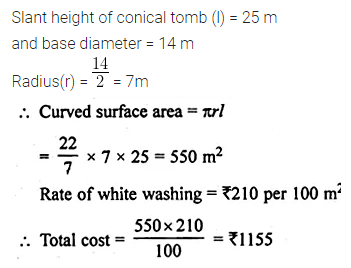Question 11.
A conical tent is 10 m high and the radius of its base is 24 m. Find:
(i) the slant height of the tent.
(ii) cost of the canvas required to make the tent, if the cost of 1 m2 canvas is Rs 70.
Solution: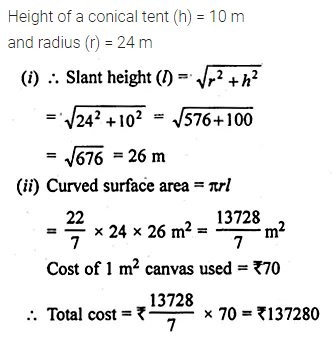Question 12.
A Jocker’s cap is in the form of a right circular cone of base radius 7 cm and height 24 cm. Find the area of the cloth required to make 10 such caps.
Solution: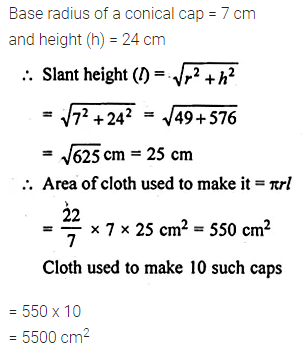Question 13.
(a) The ratio of the base radii of two right circular cones of the same height is 3 : 4. Find the ratio of their volumes.
(b) The ratio of the heights of two right circular cones is 5 : 2 and that of their base radii is 2 : 5. Find the ratio of their volumes.
(c) The height and the radius of the base of a right circular cone is half the corresponding height and radius of another bigger cone. Find:
(i) the ratio of their volumes.
(ii) the ratio of their lateral surface areas.
Solution:Question 14.
Find what length of canvas 2 m in width is required to make a conical tent 20 m in diameter and 42 m in slant height allowing 10% for folds and the stitching. Also, find the cost of the canvas at the rate of Rs 80 per metre.
Solution:Question 15.
The perimeter of the base of a cone is 44 cm and the slant height is 25 cm. Find the volume and the curved surface of the cone.
Solution: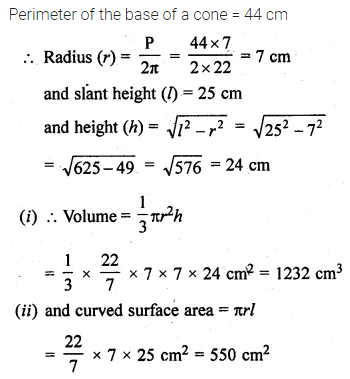Question 16.
The volume of a right circular cone is 9856 cm3 and the area of its base is 616 cm2. Find
(i) the slant height of the cone.
(ii) the total surface area of the cone.
Solution: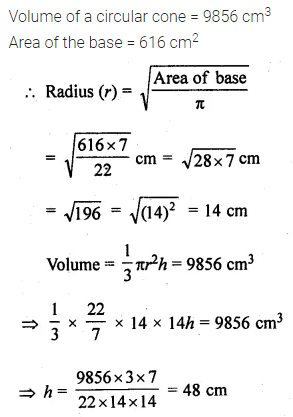Question 17.
A right triangle with sides 6 cm, 8 cm and 10 cm is revolved about the side 8 cm. Find the volume and the curved surface of the cone so formed. (Take π = 3.14)
Solution:Question 18.
The height of a cone is 30 cm. A small cone is cut off at the top by a plane parallel to its base. If its volume is $$\\ \frac { 1 }{ 27 }$$ of the volume of the given cone, at what height above the base is the section cut?
Solution: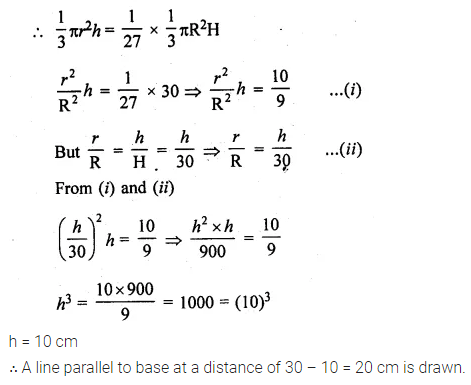Question 19.
A semi-circular lamina of radius 35 cm is folded so that the two bounding radii are joined together to form a cone. Find
(i) the radius of the cone.
(ii) the (lateral) surface area of the cone.
Solution:ML Aggarwal Class 10 Solutions for ICSE Maths